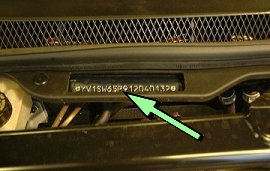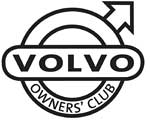# Volvo C70 S70 V70 XC70 VIN Plates

1800  |  200  |  300  |  400  |  700  |  850 - 900  |  40/50  |  60  |  70  |  80  |  90  |  XC90

This information will help you to understand the information on the VIN plate of your Volvo car.

Click on the model series number above

Example VIN Number:

YV1LW65F2Y2123456

YV1 L W 65 F 2 Y 2 123456
Manufacturer Code Vehicle Type Doors Engine Type Emission Gearbox Model Year Factory Code Chassis Number

Manufacturer Code
YV1 = cars YV2 = trucks YV3 = buses YV4 = multipurpose 4V1 = trucks 4V2 = 4V3 =
4V4 = trucks 4V5 = trucks 4V6 = 4VL = 4VM = 4VZ = MHA = PT. Central Sole Agency

Vehicle Type
G = S70, V70 BI-FUEL J = V70 BI-FUEL L = S70, V70, V70XC N = C70 S = V70, V70XC, XC70

Doors
C = 2DRS (C70 CONV) D = V70XC/XC70 ARMOURED J = V70 AWD (USA)(CDN) K = 2DRS (C70 COUPE) S = 4DRS T = 4DRS (S70 AWD) (USA)(CDN) W = 5DRS (V70) U = V70 BI-FUEL LPG V = 5DRS (V70 AWD) EXC XC (USA)(CDN) V = V70 BI-FUEL CNG Z = 5DRS (V70 XC)

Engine Type
31 = GB5252S 32 = B5244SG 33 = B5244SG2 34 = GB5252S2 41 = B5202S 43 = B5204T4 46 = B5204T2 48 = B5204T4 50 = B5234T4 51 = B5252S
52 = B5234T6 53 = B5234T3 54 = B5234S 55 = B5254FS MOT 4.4 55 = B5254S DENSO 56 = B5254T 62 = B5234T9 63 = B5244T7 56 = B5244T (To 1999) ---

Engine Type from 2000
57 = B5234T7 59 = B5234T2 60 = B5244T2 61 = B5244S 62 = B5234T8 65 = B5244S2 72 = D5252T

Engine Type from 2003
32 = B5244SG 33 = B5244SG2 49 = B5204T5 52 = B5254T4 53 = B5234T3 57 = B5234T7 58 = B5244T3 59 = B5254T2
61 = B5244S 62 = B5234T9 63 = 5244T7 64 = B5244S6 65 = B5244S2 72 = D5252T MSA 15.8 74 = D5244T2 79 = D5244T

Emissions
0 = KOD: EM A1/C1/C2/H1 2 = KOD: EM G5 3 = KOD: EM C4 4 = KOD: EM G1 6 = KOD: EM G6 7 = KOD: EM G8 8 = KOD: EM C7 9 = KOD: EM F2 A = KOD: EM J1
B = KOD: EM J4 C = KOD: EM L3 D = KOD: EM L6 E = KOD: EM N3 F = KOD: EM N5 J = KOD: EM N4 K = KOD: EM L4 L = KOD: EM L7 N = KOD: EM T1
P = KOD: EM T2 Q = KOD: EM T3 R = KOD: EM E1 T = KOD: EM T4 V = KOD: EM Z1 W = KOD: EM Z4 X = KOD: EM Z2 Y = KOD: EM Z3 ---

Gearbox
2 = M56, M59 manual gearbox 3 = M58 manual gearbox 4 = M66 manual gearbox 6 = AW42 automatic gearbox 7 = AW50AWD automatic gearbox 8 = AW42AWD automatic gearbox 9 = AW55-50SN automatic gearbox

Model Year From 1980
A = 1980 B = 1981 C = 1982 D = 1983 E = 1984 F = 1985 G = 1986 H = 1987 J = 1988 K = 1989 L = 1990 M = 1991
N = 1992 P = 1993 R = 1994 S = 1995 T = 1996 V = 1997 W = 1998 X = 1999 Y = 2000 1 = 2001 2 = 2002 3 = 2003
4 = 2004 5 = 2005 6 = 2006 7 = 2007 8 = 2008 9 = 2009 A = 2010 (code restarted) B = 2011 C = 2012 D = 2013 E = 2014 ---

Factory Code
0 = Sweden, Kalmar Plant 1 = Sweden, Torslanda Plant VCT 21(Volvo Torslandaverken) (Gothenburg) 2 = Belgium, Ghent Plant VCG 22 3 = Canada, Hatdfax Plant 4 = Italy, - Bertone models 240 5 = Malaysia, 6 = Australia,
7 = Indonesia, A = Sweden, Uddevalla Plant (Volvo Cars/TWR (Tom Walkinshaw Racing)) B = Italy, - Bertone Chongq 31 D = Italy, - Bertone models 780 E = Singapore, F = The Netherlands, Born Plant (NEDCAR) J = Sweden, Uddevalla Plant VCU 38 (Volvo Cars/ Pininfarina Sverige AB)

Engine VIN
01 = B18K 02 = B18KP 03 = B18KPD 04 = B18ES 05 = B18ED 06 = B18FTM 07 = B18KD 10 = B18U (M)-200/203 11 = B17A 12 = B18U (M)-103/113
14 = B18E/EP 15 = B18U -200/203 16 = B18U -103/113 17 = B16F 18 = B18F/FP 19 = B18FT 20 = B200 K/O 21 = B19A 22 = B200 E/O 23 = 200 E/O
24 = B19E 25 = B200 E/A 26 = B19ET 27 = B200F 28 = B200 E/E 31 = B20F 32 = B20F (M) 37 = D20 41 = B21A 44 = B21E
45 = B21F 46 = B21ET 47 = B21FT 48 = B21LH Jet 49 = B21F MPG (1981), B21F Kjet (1982) 53 = B14.4E 54 = B14.40 55 = B14.4S 61 = B27A 62 = B28A
64 = B27E 65 = B27F 68 = B28E 69 = B28F 70 = D19T (EGR) 72 = D16 74 = D24 TIC with EGR 75 = D24 TIC without EGR 76 = D24T 77 = D24 (1981-1984)
79 = D19T 80 = B230G 81 = B23A 82 = B230GT & B230FD 83 = B230FD w/ pulsair 84 = B23E 85 = B230FB 86 = B230FT - EGR 87 = B230FT - non EGR 88 = B23F (1983-1984), B230F (1985-95)
89 = B234F 92 = B6244F 93 = B6254F 95 = B6304F with air pump 96 = B6204F without air pump 98 = N6304G 99 = B6254G --- --- ---

### C70

Location of the Chassis/VIN Plate
On the nearside inner wing on a metal plate held by two silver rivets.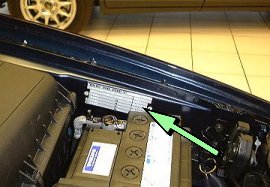Visible VIN
Viewed through the nearside base of the front windscreen on an embossed plate held by two rivets.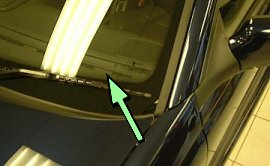Stamped Chassis Number
Stamped onto the centre of the bulkhead facing up.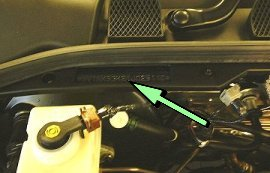### S70

Location of Chassis/VIN Plate
On the offside wing facing up on a metal plate held by two black rivets. On the S 70 & S 80 it is on the nearside wing. Later models it has been located on the Right B pillar just under the rear door hinge in a black sticker format.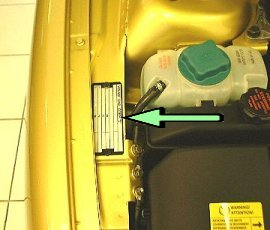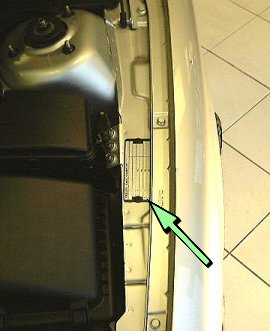Visible VIN
Viewed through the nearside base of the front windscreen on a embossed plate held by two Volvo rivets.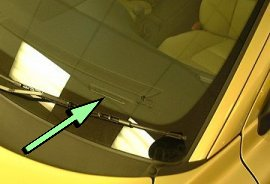### V70

Location of Chassis/VIN Plate
On the offside wing on a metal plate held by two black rivets.

Chassis/VIN Plate
On the offside wing on a metal plate held by two black rivets.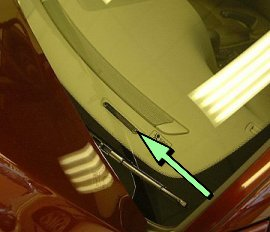Stamped Chassis Number
Stamped into the centre of the bulkhead facing up.